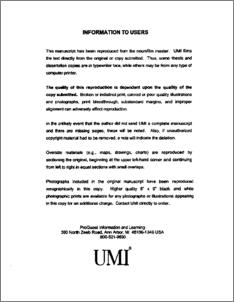# Some exact and approximate inverse boundary and scattering problems

Masood, Khalid (2002) Some exact and approximate inverse boundary and scattering problems. PhD thesis, King Fahd University of Petroleum and Minerals.Preview
PDF
3282Kb

-

## English Abstract

The inverse problems are important in seismic exploration and underground geology. The frequently used earth model for such a purpose is that of a homogeneous and isotropic medium. This may not be an accurate description of many practical situations. We consider a model that incorporates the effects of damping in the medium and develop an inversion procedure for this case. It is hoped that the results based upon this model will prove to be more realistic in some situations of interest. We consider the inverse problem of recovering the initial disturbance from the information of the final data. The damped and undamped models are compared by performing some numerical experiments. An application of damped wave equation is also presented which involve the introduction of a damping parameter in such a way that it closely approximates the heat conduction model. It is shown that the modified model based on the damped wave equation behaves much better than the classical heat conduction model. The Gelfand-Levitan and Marchenko (GLM) procedure is used for the development of inversion formalism for estimating parameter changes across inhomogeneities. We use this method to recover a one-dimensional shear velocity variation in the surface of the earth. The Love waves, traveling in a layer overlying a half space, incident upon delta function potential, are considered. The equation of motion for Love waves is transformed to the Schrodinger equation by assuming a small variation in shear velocity which leads to the potential term in the governing equation of Love waves. We consider a problem of the identification of physical properties of the earth using the damped wave equation based on the linearized inversion associated with Born's inversion theory. We assume that damping and sound speed are well approximated by the background plus the perturbation. The application of the method leads to a linear integral equation involving variations in sound speed and damping. Our aim is to recover these variations in velocity and damping, which in turn yields a map of the interfaces in the interior of the earth.

Item Type: Thesis (PhD) March 2002 Completion Math College Of Sciences > Mathematical Science Dept Masood, Khalid Zaman, Fiazuddin Korvin, Gabor and Amin, Alaaeldin A. M. and Furati, Khaled M. and Messaoudi, Salim 10151 KFUPM ePrints Admin 22 Jun 2008 16:57 25 Apr 2011 10:23

Repository Staff Only: item control page